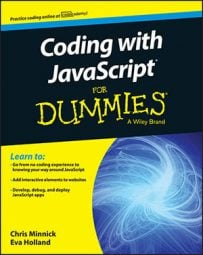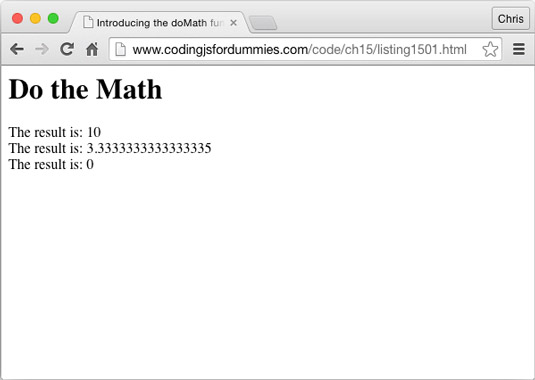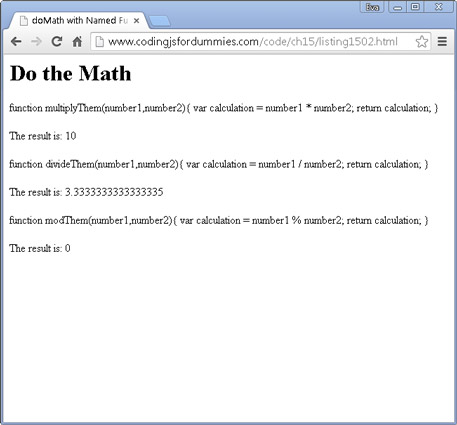##### Coding with JavaScript For DummiesJavaScript functions are objects. This statement is the key to understanding many of the more advanced JavaScript topics, including callback functions. Functions, like any other object, can be assigned to variables, be passed as arguments to other functions, and created within and returned from functions.

## Passing functions as arguments

A callback function is a function that is passed as an argument to another function. Callback functions are a technique that’s possible in JavaScript because of the fact that functions are objects.

Function objects contain a string with the code of the function. When you call a function by naming the function, followed by ( ), you’re telling the function to execute its code. When you name a function or pass a function without the ( ), the function does not execute.

With some examples of callback functions you can use the addEventListener method, such as

`document.addEventListener(‘click’,doSomething,false);`

This method takes an event (click) and a Function object (doSomething) as arguments. The callback function doesn’t execute right away. Instead, the addEventListener method executes the function when the event occurs.

## Writing functions with callbacks

Here’s a simple example function, doMath, that accepts a callback function as an argument:

```function doMath(number1,number2,callback) {
var result = callback(number1,number2);
document.write ("The result is: ": + result);
}```

This function is a generic function for returning the result of any math operation involving two operands. The callback function that you pass to it specifies what actual operations will be done.

To call our doMath function, pass two number arguments and then a function as the third argument:

```doMath(5,2,function(number1,number2){
var calculation = number1 * number2 / 6;
return calculation;
});```

This is a complete web page that contains the doMath function and then invokes it several times with different callback functions.

```<html>
<title>Introducing the doMath function</title>
<script>
function doMath(number1,number2,callback){
var result = callback(number1,number2);
document.getElementById("theResult").innerHTML += ("The result is: " + result + "<br>");
}

doMath(5,2,function(number1,number2){
var calculation = number1 * number2;
return calculation;
});
doMath(10,3,function(number1,number2){
var calculation = number1 / number2;
return calculation;
});
doMath(81,9,function(number1,number2){
var calculation = number1 % number2;
return calculation;
});
}, false);
</script>
<body>
<h1>Do the Math</h1>
<div id="theResult"</div>
</body>
</html>```

The result of running this code in a browser is shown here.Doing calculations using callbacks.

## Using named callback functions

In the examples above, the callback functions were all written as anonymous functions. It’s also possible to define named functions and then pass the name of the function as a callback function.

Anonymous functions are functions that you create without giving them names.

Using named functions as callbacks can reduce the visual code clutter that can come with using anonymous functions. Here is an example of how to use a named function as a callback. This example also features the following two improvements:

• A test has been added to the doMath function to make sure that the callback argument is actually a function.

• It prints out the code of the callback function before it displays the result of running it.

```<html>
<title>doMath with Named Functions</title>
<script>
function doMath(number1,number2,callback){
if (typeof callback === "function") {
var result = callback(number1,number2);
document.getElementById("theResult").innerHTML += (callback.toString() + "<br><br>The result is: " + result + "<br><br>");
}
}
function multiplyThem(number1,number2){
var calculation = number1 * number2;
return calculation;
}
function divideThem(number1,number2){
var calculation = number1 / number2;
return calculation;
}
function modThem(number1,number2){
var calculation = number1 % number2;
return calculation;
}
doMath(5,2,multiplyThem);
doMath(10,3,divideThem);
doMath(81,9,modThem);
}, false);
</script>
<body>
<h1>Do the Math</h1>
<div id="theResult"</div>
</body>
</html>```

This is the result of running this code in a browser.Doing math with named callbacks.

Using named functions for callbacks has two advantages over using anonymous functions for callbacks: